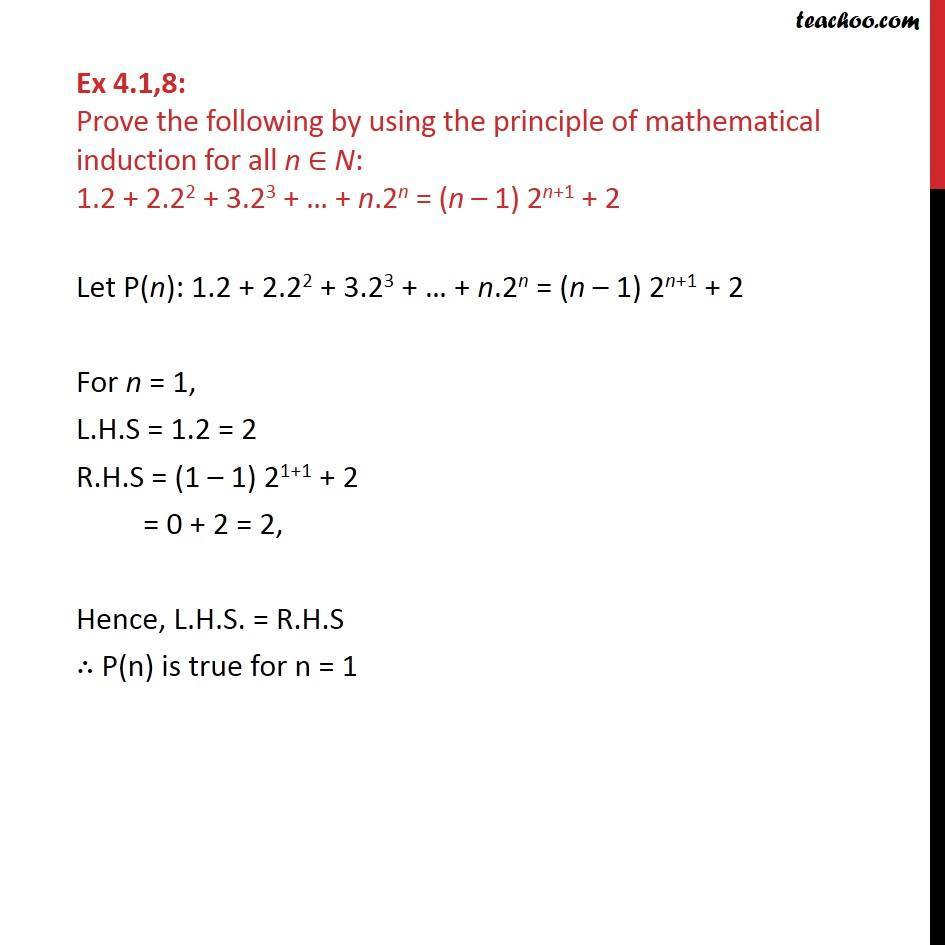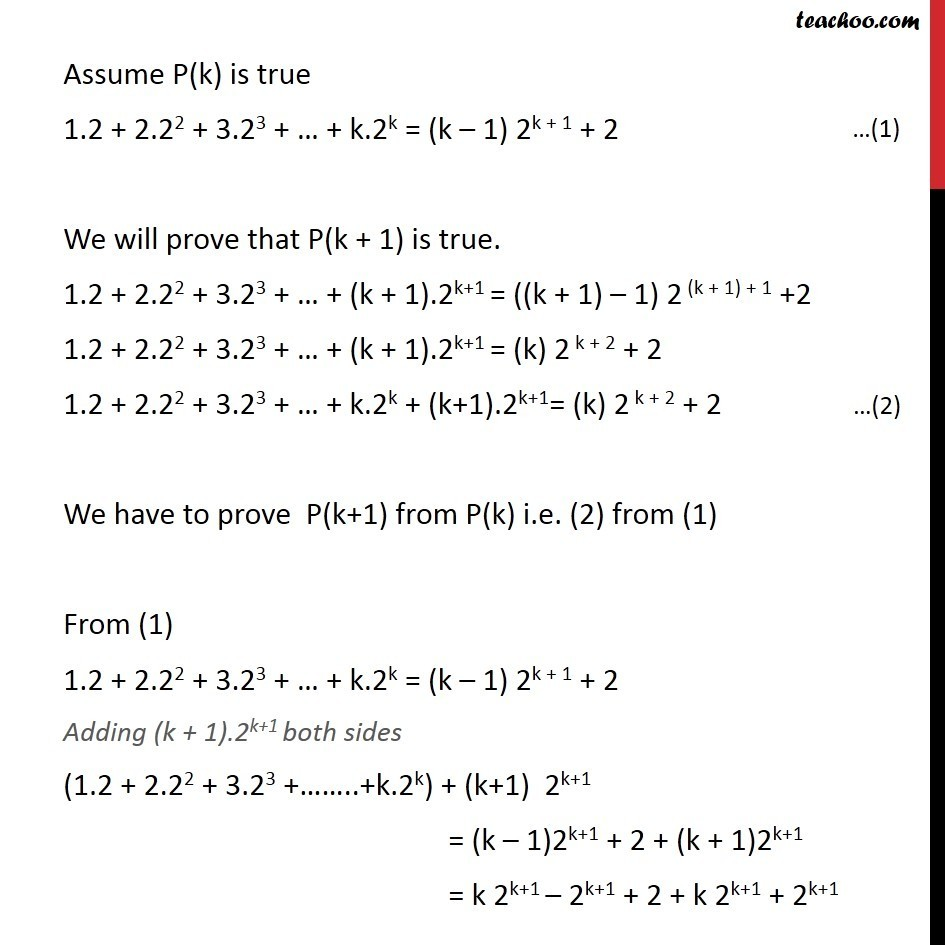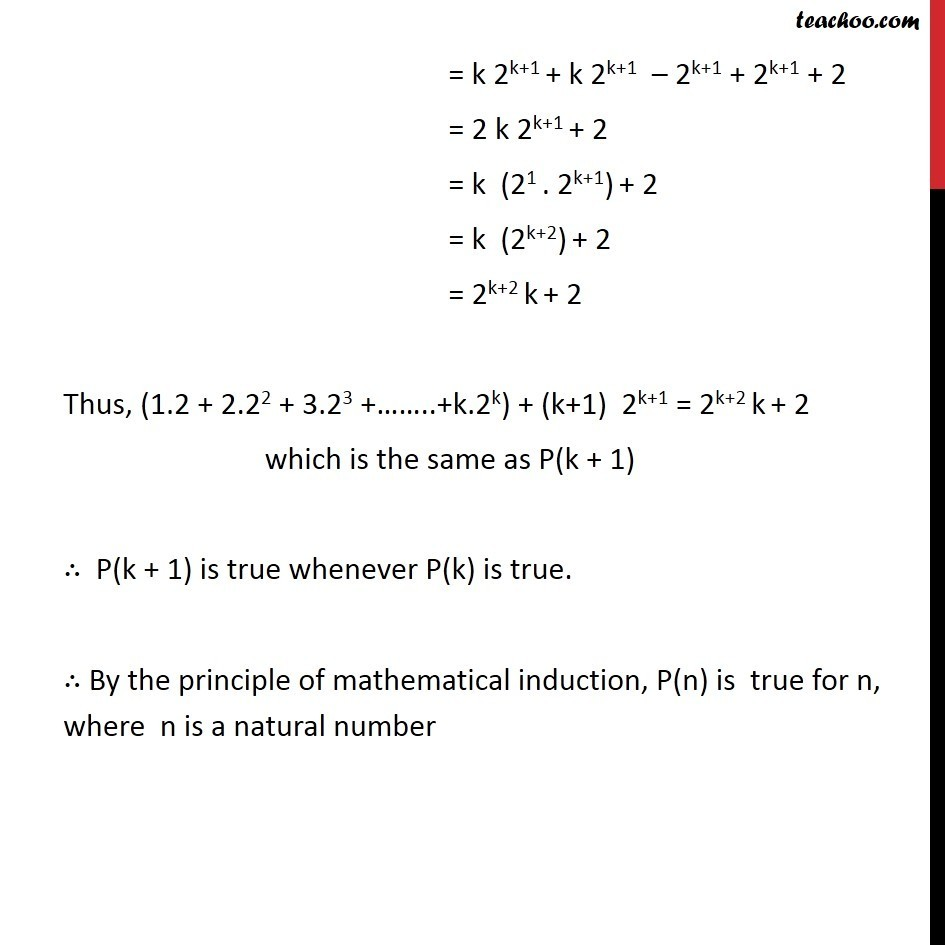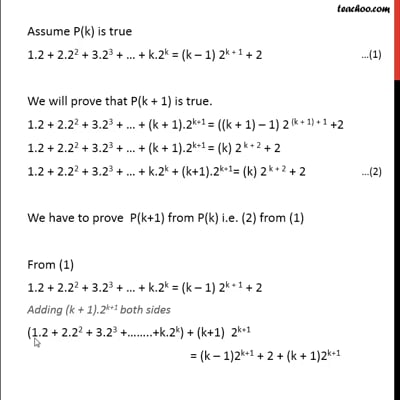Chapter 4 Class 11 Mathematical Induction
Concept wiseThis video is only available for Teachoo black users

Introducing your new favourite teacher - Teachoo Black, at only ₹83 per month

### Transcript

Ex 4.1,8: Prove the following by using the principle of mathematical induction for all n ∈ N: 1.2 + 2.22 + 3.23 + … + n.2n = (n – 1) 2n+1 + 2 Let P(n): 1.2 + 2.22 + 3.23 + … + n.2n = (n – 1) 2n+1 + 2 For n = 1, L.H.S = 1.2 = 2 R.H.S = (1 – 1) 21+1 + 2 = 0 + 2 = 2, Hence, L.H.S. = R.H.S ∴ P(n) is true for n = 1 Assume P(k) is true 1.2 + 2.22 + 3.23 + … + k.2k = (k – 1) 2k + 1 + 2 We will prove that P(k + 1) is true. 1.2 + 2.22 + 3.23 + … + (k + 1).2k+1 = ((k + 1) – 1) 2 (k + 1) + 1 +2 1.2 + 2.22 + 3.23 + … + (k + 1).2k+1 = (k) 2 k + 2 + 2 1.2 + 2.22 + 3.23 + … + k.2k + (k+1).2k+1= (k) 2 k + 2 + 2 We have to prove P(k+1) from P(k) i.e. (2) from (1) From (1) 1.2 + 2.22 + 3.23 + … + k.2k = (k – 1) 2k + 1 + 2 Adding (k + 1).2k+1 both sides (1.2 + 2.22 + 3.23 +……..+k.2k) + (k+1) 2k+1 = (k – 1)2k+1 + 2 + (k + 1)2k+1 = k 2k+1 – 2k+1 + 2 + k 2k+1 + 2k+1 = k 2k+1 + k 2k+1 – 2k+1 + 2k+1 + 2 = 2 k 2k+1 + 2 = k (21 . 2k+1) + 2 = k (2k+2) + 2 = 2k+2 k + 2 Thus, (1.2 + 2.22 + 3.23 +……..+k.2k) + (k+1) 2k+1 = 2k+2 k + 2 which is the same as P(k + 1) ∴ P(k + 1) is true whenever P(k) is true. ∴ By the principle of mathematical induction, P(n) is true for n, where n is a natural number